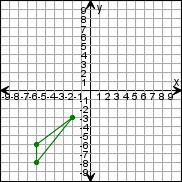Create a new printableGeometry
Math Worksheets

Sample - Click above to make a new math worksheet (PDF).
 Name _____________________________Date ___________________
Transformations
Draw the triangle after the transformations.

 1 * This is a pre-made sheet.Use the link at the top of the page for a printable page.
 2 Rotate the triangle 180clockwise around (0,0).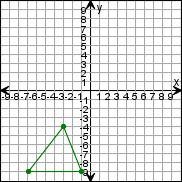3 Reflect the triangle over the y-axis.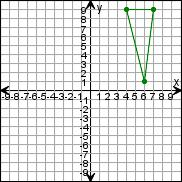4 Rotate the triangle 180clockwise around (0,0).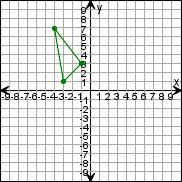5 Reflect the triangle over the x-axis.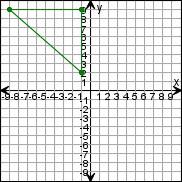6 Translate the triangle down 5 units, then 6 units left.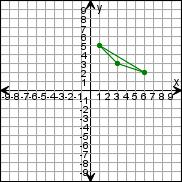7 Reflect the triangle over the x-axis.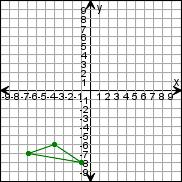8 Translate the triangle 6 units right.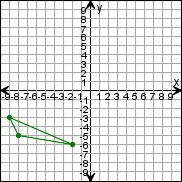9 Rotate the triangle 270clockwise around (0,0).